SLPS306A May   2012  – September 2015

PRODUCTION DATA.

1. 1Features
2. 2Applications
3. 3Description
4. 4Revision History
5. 5Specifications
6. 6Device and Documentation Support
7. 7Mechanical, Packaging, and Orderable Information

• YZB|4

## 5 Specifications

### 5.1 Electrical Characteristics

TA = 25°C (unless otherwise noted)
PARAMETER TEST CONDITIONS MIN TYP MAX UNIT
STATIC CHARACTERISTICS
BVDSS Drain-to-source voltage VGS = 0 V, ID = 250 μA 12 V
IDSS Drain-to-source leakage current VGS = 0 V, VDS = 9.6 V 1 μA
IGSS Gate-to-source leakage current VDS = 0 V, VGS = 8 V 100 nA
VGS(th) Gate-to-source threshold voltage VDS = VGS, ID = 250 μA 0.65 0.8 1.1 V
RDS(on) Drain-to-source on resistance VGS = 1.8 V, ID = 1 A 38 53
VGS = 2.5 V, ID = 1 A 29 39
VGS = 4.5 V, ID = 1 A 26 34
gfs Transconductance VDS = 6 V, ID = 1 A 23 S
DYNAMIC CHARACTERISTICS
CISS Input capacitance VGS = 0 V, VDS = 6 V, ƒ = 1 MHz 385 462 pF
COSS Output capacitance 245 294 pF
CRSS Reverse transfer capacitance 18.1 22.6 pF
Rg Series gate resistance 3 Ω
Qg Gate charge total (4.5 V) VDS = 6 V, ID = 1 A 2.3 2.9 nC
Qgd Gate charge gate-to-drain 0.3 nC
Qgs Gate charge gate-to-source 0.5 nC
Qg(th) Gate charge at Vth 0.3 nC
QOSS Output charge VDS = 6.0 V, VGS = 0 V 1.8 nC
td(on) Turn on delay time VDS = 6 V, VGS = 4.5 V, ID = 1 A
RG = 20 Ω
3.9 ns
tr Rise time 5.9 ns
td(off) Turn off delay time 14.4 ns
tf Fall time 9.7 ns
DIODE CHARACTERISTICS
VSD Diode forward voltage IS = 1 A, VGS = 0 V 0.7 1 V
Qrr Reverse recovery charge VDS= 6 V, IS = 1 A, di/dt = 100 A/μs 2.4 nC
trr Reverse recovery time 11.5 ns

### 5.2 Thermal Information

(TA = 25°C unless otherwise stated)
THERMAL METRIC MIN TYP MAX UNIT
RθJA Thermal resistance junction-to-ambient (minimum Cu area) 228.6 °C/W
RθJA Thermal resistance junction-to-ambient (1 in2 Cu area) 131.1 °C/WMax RθJA = 131.1°C/W when mounted on 1 inch2 of 2 oz. Cu.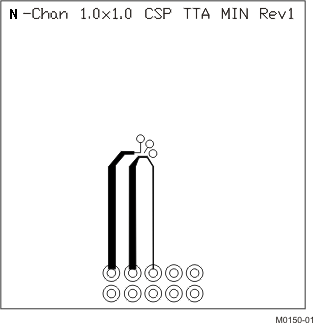Max RθJA = 228.6°C/W when mounted on minimum pad area of 2 oz. Cu.

### 5.3 Typical MOSFET Characteristics

TA = 25°C (unless otherwise noted)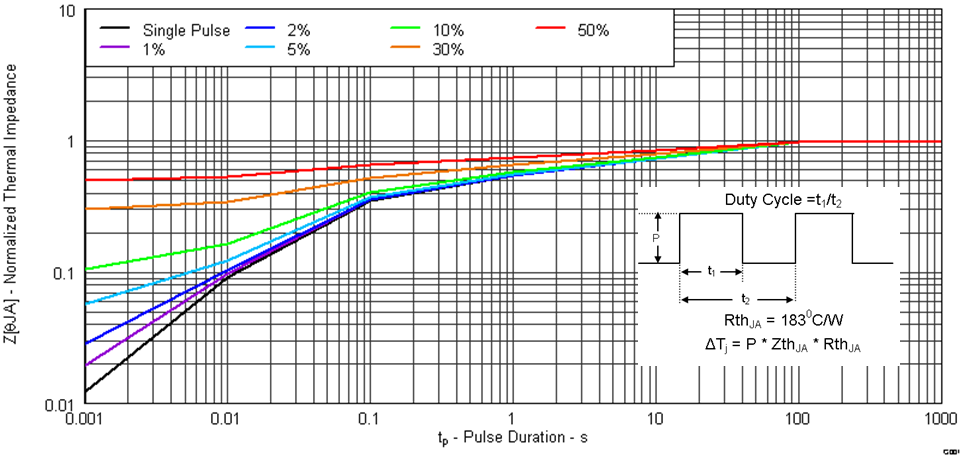Figure 1. Transient Thermal Impedance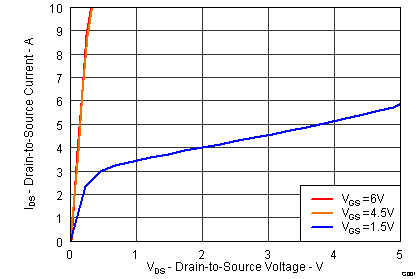Figure 2. Saturation Characteristics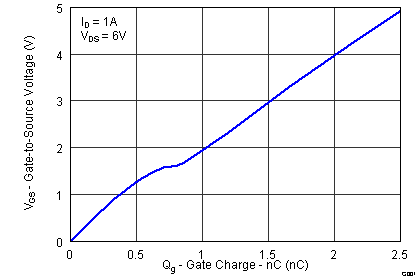Figure 4. Gate Charge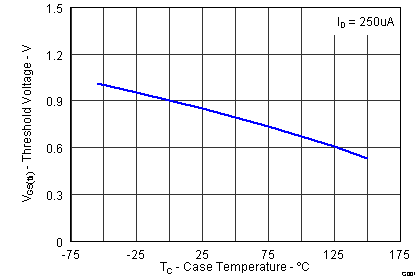Figure 6. Threshold Voltage vs TemperatureFigure 8. On Resistance vs Temperature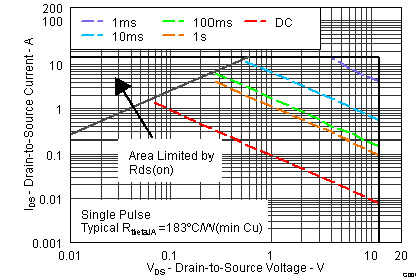Figure 10. Maximum Safe Operating Area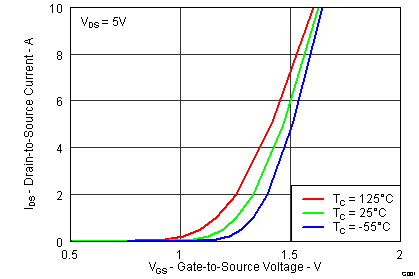Figure 3. Transfer CharacteristicsFigure 5. Capacitance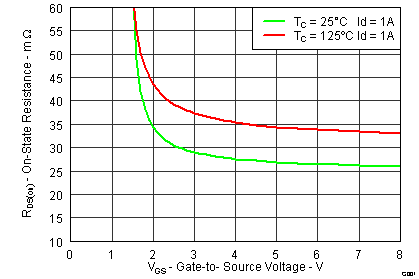Figure 7. On Resistance vs Gate Voltage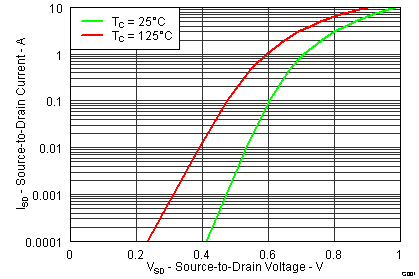Figure 9. Typical Diode Forward Voltage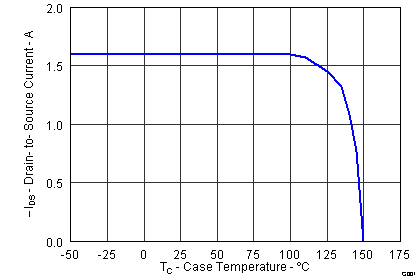Figure 11. Maximum Drain Current vs Temperature